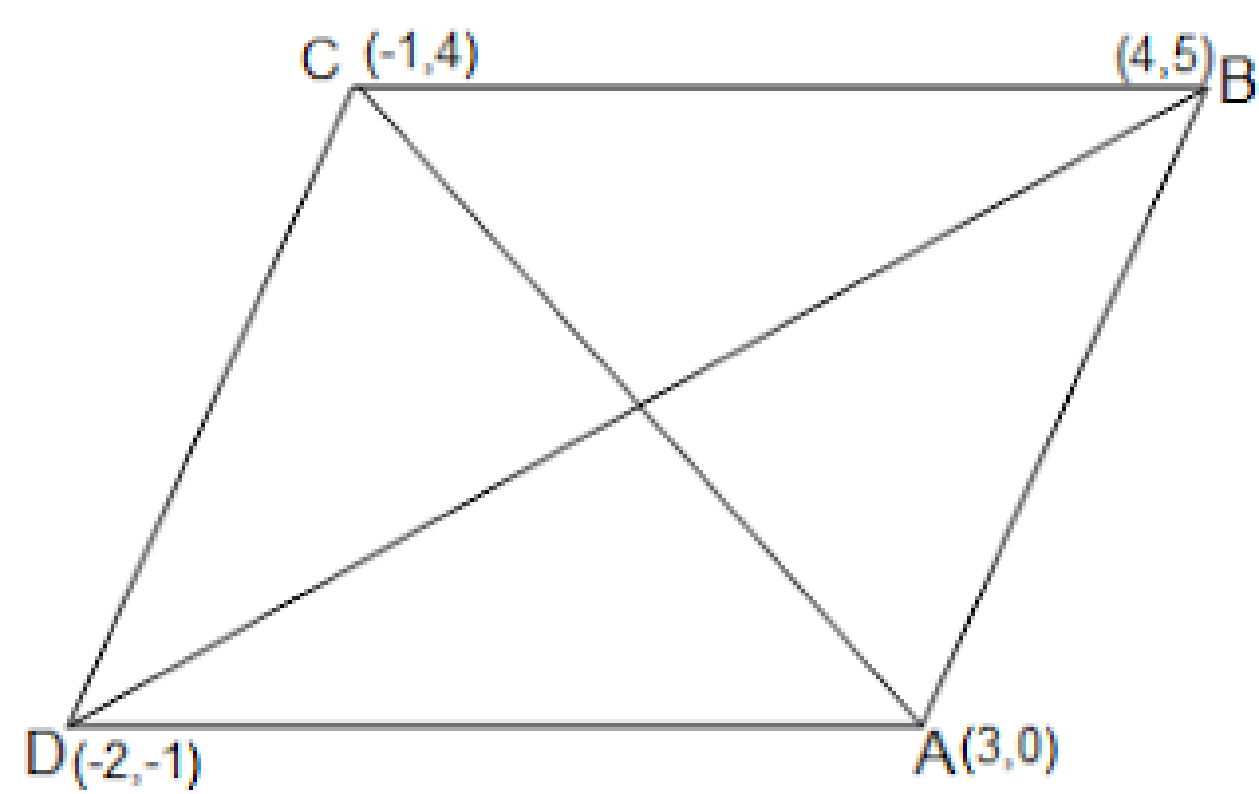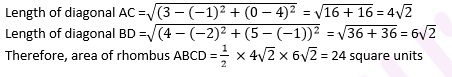# NCERT Solutions For Class 10 MATH Chapter – 7 Exercise – 7.2

NCERT Solutions For Class 10 Math Chapter- 7 Exercise – 7.2

Q1. Find the coordinates of the point which divides the join of (-1 , 7) and (4 , -3) in the ratio 2:3

Solution:-

X = (2×4 + 3x(-1)/(2+3) = 8-3)/5 = 1

Y = (2 x -3 + 3 x 7)/(2+3) = (-6 + 21)/5 = 3

Therefore , the point is (1,3)

Q2. Find the coordinates of the points of trisection of the line segment joining (4,-1) and (-2,-3).

Solution:-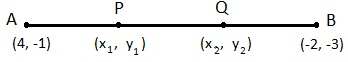P divides AB internally in the ratio 1:2.

X1 = (1x(-2) + 2 x 4)/3  = 2

Y1 =

(1x(-3) + 2(-1)/(1+2) = -5/3

P(X1 , Y1) = p(2 , -5/3)

Point Q divides AB internally in the ratio 2:1

X2 = (2x(-2) + 1 x4)/(2 +1) = 0

Y2 = (2x(-3) + 1x(-1)/(2+1) = -7/3

The coordinate of the point Q is (0 , -7/3)

Q3. To conduct Sports Day activities in your rectangular shaped school ground ABCD lines have been drawn with chalk powder at a distance 1 m each 100 flower pots have been placed at a distance of 1 m from each other along AD as shown in the following figure Niharika runs 1/4th the distance AD on the 2nd line and posts a green flag. Preet runs 1/5th the distance AD on the eights line and posts a red flag. What is the distance between the both flags ? If Rashmi has to post a blue flag exactly halfway between the line segment joining the two flags,where should she post her flag ?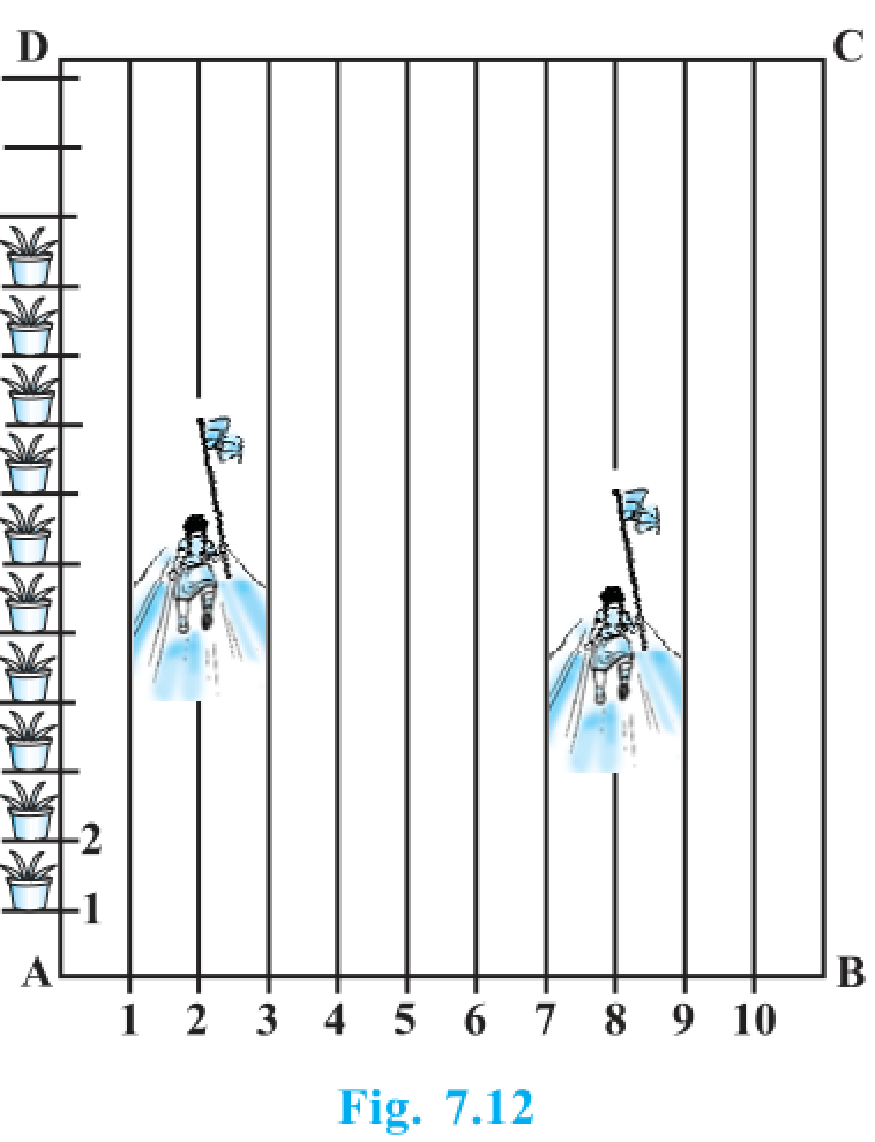Solution:-

A (2,25)  B(8,20)

AB = √(8-2)2 + (20-25)2

= √61 m.

X = (2+8)/2 = 5

Y = (20 + 25)/2 = 45/2

P(x,y) = (5,45/2)

Q4. Find the ratio in which the line segment joining the points (-3,10) and (6,-8) is divided by (-1,6).

Solution:-

-1 = (6k-3) (k+1)

-k – 1 = 6k – 3

K = 2/7

Therefore , the required ratio is 2:7 .

Q5.  Find the ratio in which the the line segment joining A (1 , -5) and B (-4 ,5) is divided by the x – axis Also  find the coordinates of the point of division.

Solution:-

P(x,y) = (-4(1) + 1)/(1+1)

= (5(1) -5)/(1+1)

= (-3/2 , 0 )

Q6. If (1,2) , (4,y) , (x,6) and (3,5) are the vertices of a parallelogram taken in order find x and y.

Solution:-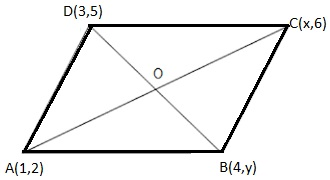Midpoint of AC = (1+X)/2 , (2+6)/2) = (1+X)/2,4)

Midpoint  of AC and BD are same , this implies

(1+x)/2 = 7/2 and 4 = (5+y)/2

X = 6 and y = 3

1. Find the coordinates of a point A, where AB is the diameter of circle whose centre is (2, – 3) and B is (1, 4).

Solution:

Let the coordinates of point A be (x, y).

Mid-point of AB is (2, – 3), which is the centre of the circle.

Coordinate of B = (1, 4)

(2, -3) =((x+1)/2 , (y+4)/2)

(x+1)/2 = 2 and (y+4)/2 = -3

x + 1 = 4 and y + 4 = -6

x = 3 and y = -10

The coordinates of A(3,-10).

1. If A and B are (-2, -2) and (2, -4), respectively, find the coordinates of P such that AP = 3/7 AB and P lies on the line segment AB.

Solution: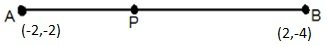The coordinates of point A and B are (-2,-2) and (2,-4) respectively. Since AP = 3/7 AB

Therefore, AP: PB = 3:4

Point P divides the line segment AB in the ratio 3:4.

1. Find the coordinates of the points which divide the line segment joining A (- 2, 2) and B (2, 8) into four equal parts.

Solution: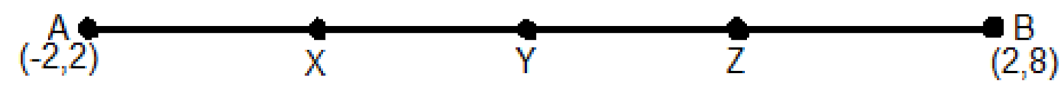Draw a figure, line dividing by 4 points.

From the figure, it can be observed that points X, Y, Z are dividing the line segment in a ratio 1:3, 1:1, 3:1 respectively.

1. Find the area of a rhombus if its vertices are (3, 0), (4, 5), (-1, 4) and (-2,-1) taken in order.

[Hint: Area of a rhombus = 1/2 (product of its diagonals)

Solution:

Let A(3, 0), B (4, 5), C( – 1, 4) and D ( – 2, – 1) are the vertices of a rhombus ABCD.